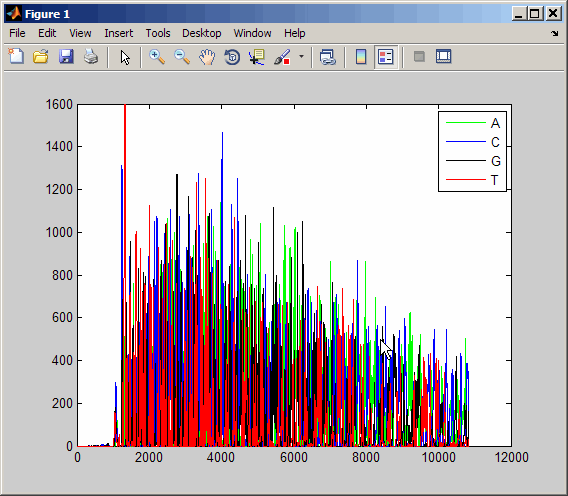traceplot

Draw nucleotide trace plots

Syntax

traceplot(TraceStructure)
traceplot(A, C, G, T)
h = traceplot(...)

Description

traceplot(TraceStructure) creates a trace plot from data in a structure with fields A, C, G, and T.

traceplot(A, C, G, T) creates a trace plot from data in vectors A, C, G, and T.

h = traceplot(...) returns a structure with the handles of the lines corresponding to A, C, G, T.

Examples

1. Read trace data from an SCF-formatted file into a MATLAB® structure.

tstruct =

A: [10827x1 double]
C: [10827x1 double]
G: [10827x1 double]
T: [10827x1 double]
2. Draw a nucleotide trace plot of the data.

traceplot(tstruct)